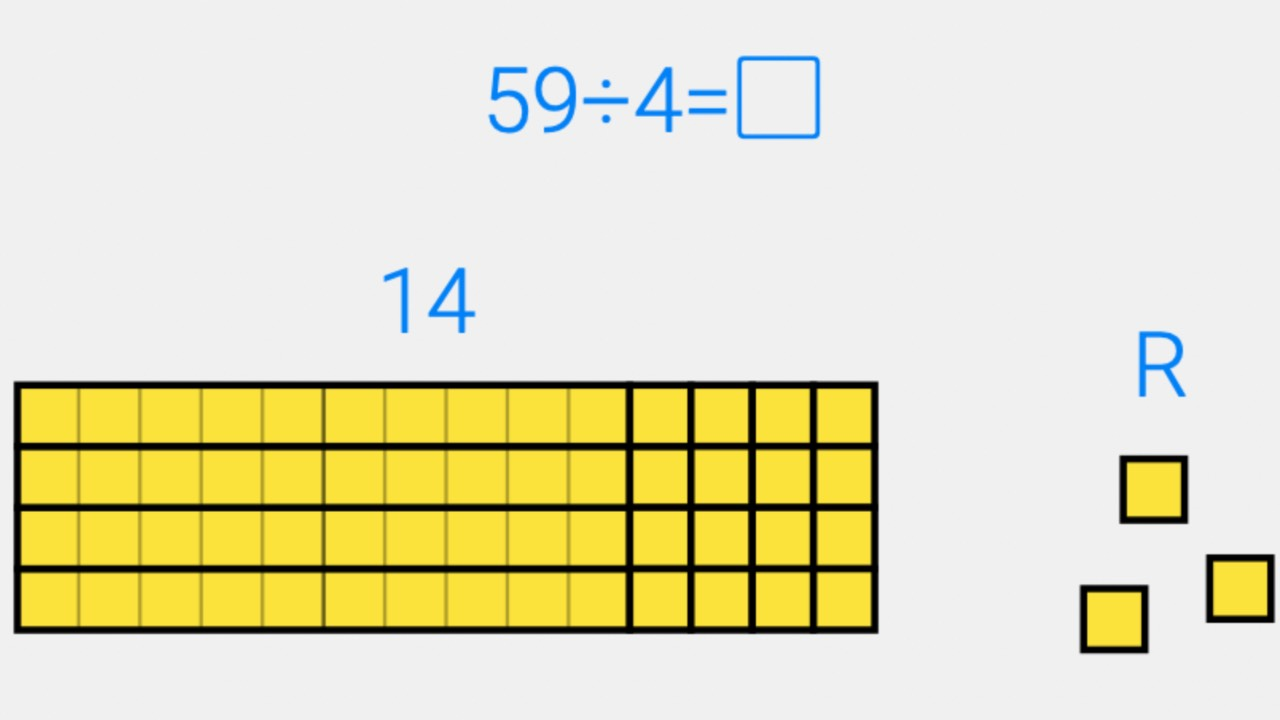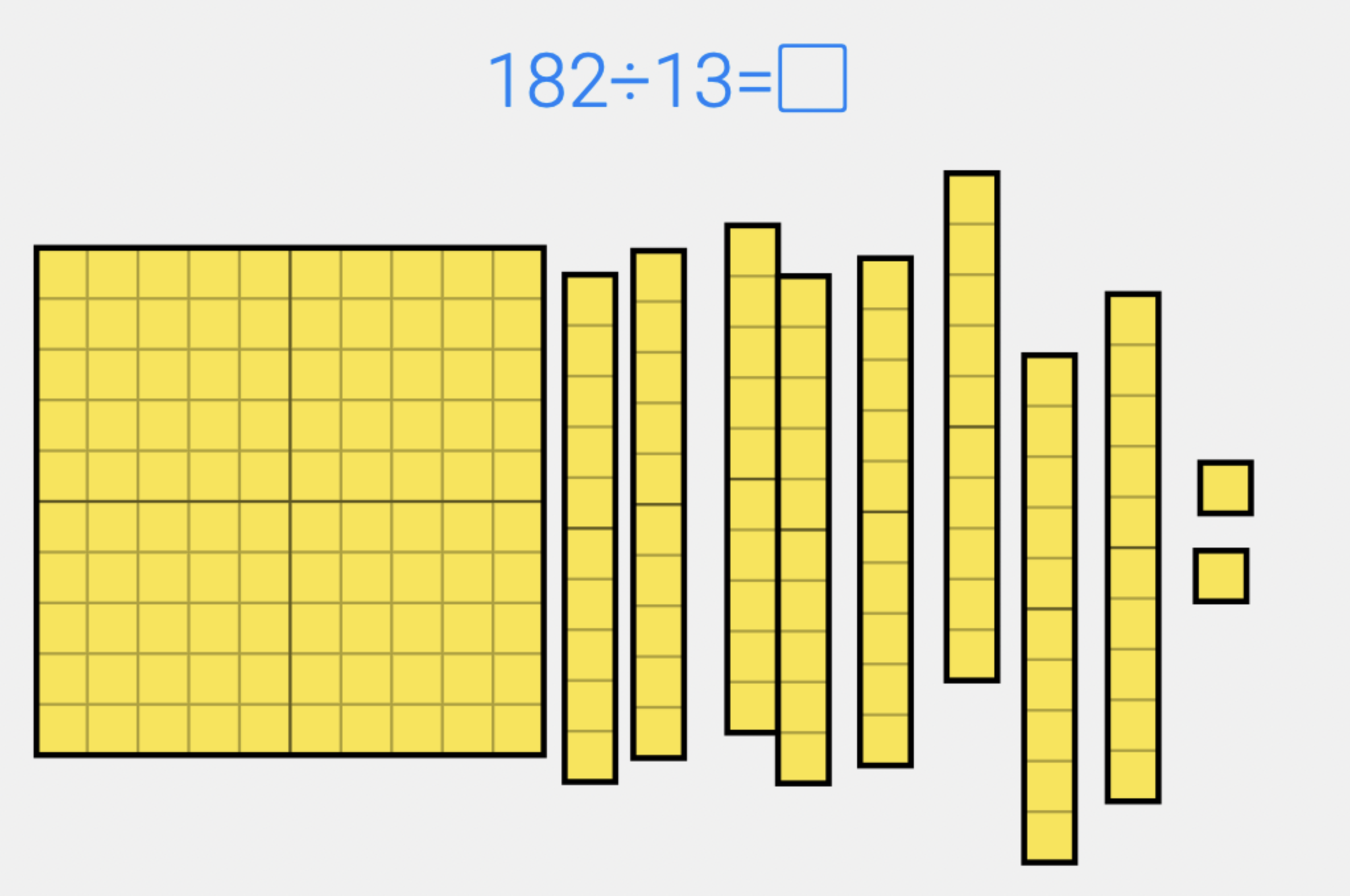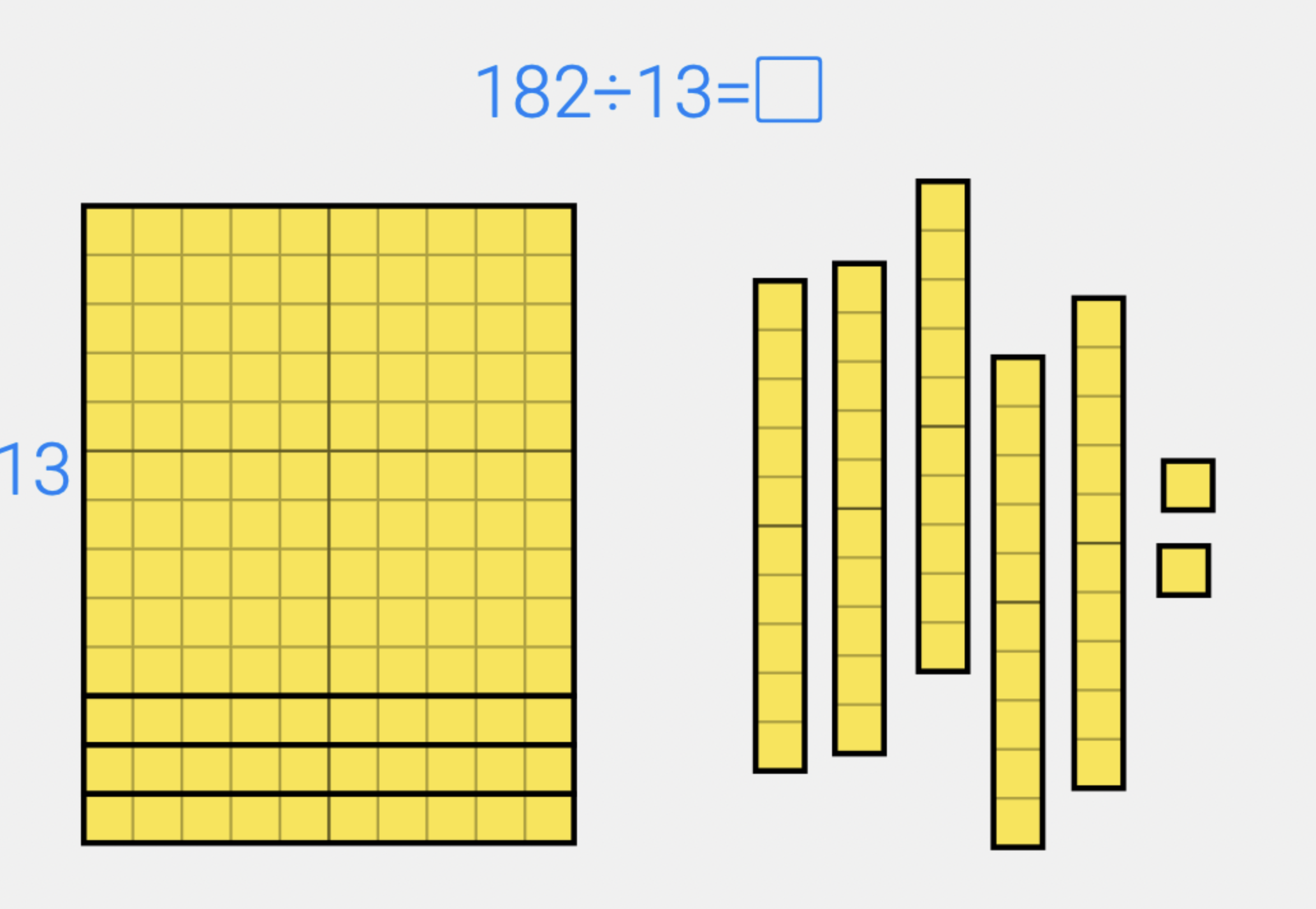# Using Arrays for Division

Aug 04, 2022Now that you have learned the importance of arrays and learned how to build them for one digit and two digit numbers, let’s take a look at how arrays can also help support students’ understanding of division.

When we build a multiplication array, we are showing the factors through the length and width.  We are showing the product with the total number of squares in our array.  To solve a division problem, we work in reverse.  We start with our dividend or the total number.  Then we can organize those pieces into rows based on our divisor.  Then, once we have passed out all the pieces, we will know how many columns we have and we will know our quotient.

## Division Array Model

Take a look at how that works, step by step.  We will consider the problem 182 ÷ 13. We'll start by getting out 182 pieces.Since our divisor is 13 we want to start arranging our pieces into 13 rows. We don't yet know how many columns we will need. Once we have 13 rows, we can use the extra pieces to start making our columns. We will keep adding columns until we have used up all the pieces and they are in the shape of a square or a rectangle.## Division Arrays With Remainders

What about remainders? We may reach a point in building our array where there are some pieces left over. In this case there are not enough to create a new column. These pieces are our remainder.

In the example of 59÷4 we have 59 squares that we need to arrange into four rows. After creating our four rows we have 19 squares left. We can put 16 of them into four new columns but we still will have 3 left over. This is not enough to create a whole new column so this is our remainder.